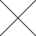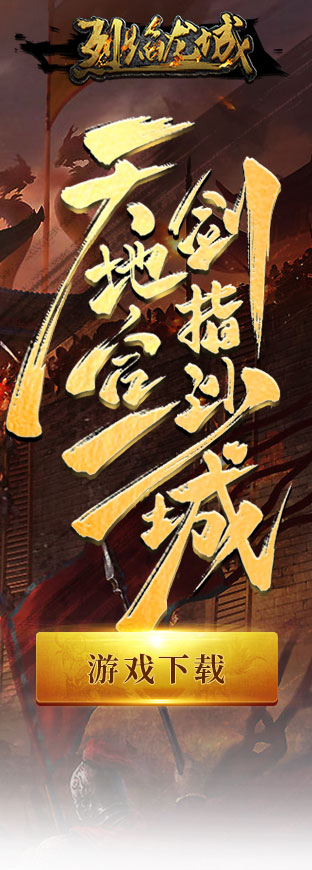# 仙侠记非R玩家吉星高照刷6吉星全攻略

2011-12-08  00:00:00

1次投掷6个骰子出0个、1个、2个、3个、4个、5个、6个吉星的情况。

1、投掷出0个吉星的概率为：P0=（5/6）^6=0.3349;^：代表是幂。

2、投掷出1个吉星的概率为：P1=6*（1/6）*（（5/6）^5）=0.4019

3、投掷出2个吉星的概率为：P2=（（6*5）/2*1）*（（1/6） ^2）*（（5/6）^4）=0.2009

4、投掷出3个吉星的概率为：P3=（（6*5*4）/3*2*1）*（（1/6） ^3）*（（5/6）^3）=0.05358

5、投掷出4个吉星的概率为：P4=（（6*5*4*3）/4*3*2*1）*（（1/6） ^4）*（（5/6）^2）=0.0080

6、投掷出5个吉星的概率为：P5=6*（5/6）*（（1/6）^5）=0.00006

7、投掷出6个吉星的概率为：P6=（1/6）^6=0.0000021

10个1=0.4019 ^10=0.00001

1个0，9个1=10*0.3349*0.4019^9=0.0000916

2个0，8个1=45*（0.3349^2）*（0.4019^8）=0.00343

3个0，7个1=120*（0.3349^3）*（0.4019^7）=0.00763

4个0，6个1=210*（0.3349^4）*（0.4019^6）=0.0111

5个0，5个1=252*（0.3349^5）*（0.4019^5）=0.0111

6个0，4个1=210*（0.3349^6）*（0.4019^4）=0.0076

7个0，3个1=120*（0.3349^7）*（0.4019^3）=0.0036

8个0，2个1=45*（0.3349^8）*（0.4019^2）=0.0011

9个0，1个1=10*（0.3349^9）*（0.4019^1）=0.000021

10个0，=0.3349^10=0.0000018

【仙峰游戏】以上就是本文全部内容，更多相关攻略，敬请关注仙峰游戏。

### 猜你喜欢

•聊斋搜灵录

新国风御灵志怪手游

立即下载
•英雄爱三国

九宫格休闲套路手游

立即下载
•烈焰龙城

复古76攻城手游

立即下载
•情剑奇缘

轻策略武侠RPG

立即下载
•烈火雷霆

官方正版传奇

立即下载

### 热门推荐

X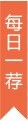烈焰龙城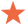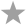(0.0分)

角色扮演 | 复古76攻城手游

扫一扫下载游戏